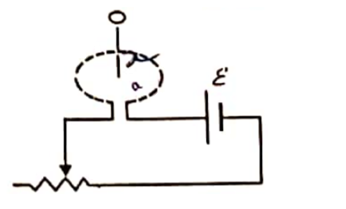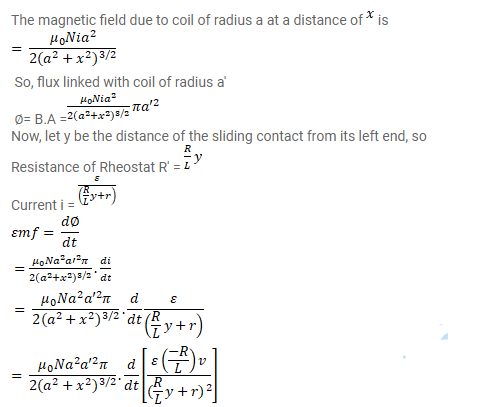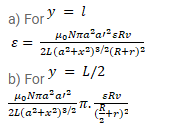# Figure shows a circular coil of N turns and radius a,

Question:

Figure shows a circular coil of $\mathrm{N}$ turns and radius a, connected to a battery of emf $\varepsilon$ through a rheostat. The rheostat has a total length $\mathrm{L}$ and resistance $\mathrm{R}$. The resistance of the coil is $\mathrm{r}$. A small circular loop of radius $a^{\prime}$ and resistance $r^{\prime}$ is placed coaxially with the coil. The centre of the loop is at a distance $x$ from the centre of the coil. In the beginning the sliding contact of the rheostat is at the left end and then onwards it is moved towards right at a constant speed v. Find the emf induced in the small circular loop at the instant(a) the contact begins to slide and

(b) it has slid through half the length of the rheostat.

Solution: